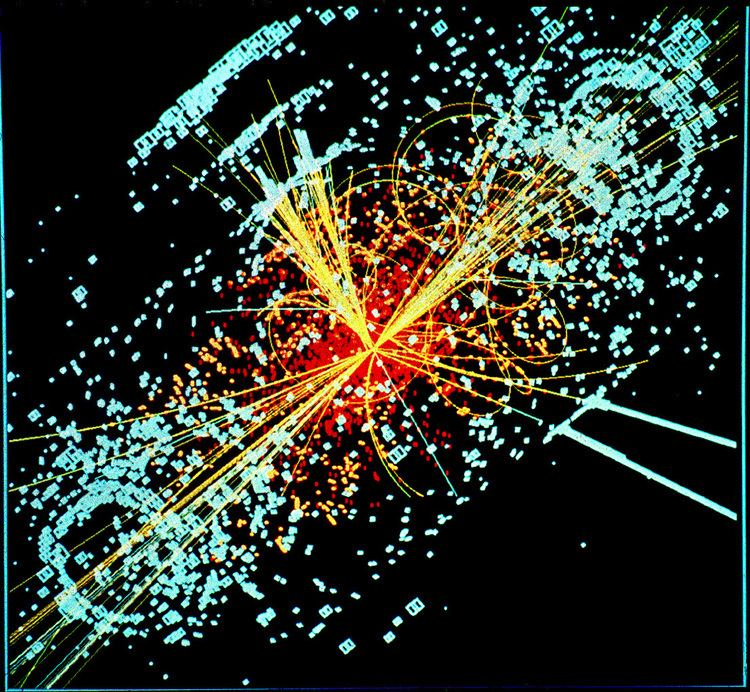# Cosmological constant problem

Updated on
Covid-19In cosmology, the cosmological constant problem is the disagreement between measured values of the vacuum energy density (the small value of the cosmological constant) and the zero-point energy suggested by quantum field theory.

Depending on the assumptions, the discrepancy ranges from 40 to more than 100 orders of magnitude, a state of affairs described by Hobson et al. (2006) as "the worst theoretical prediction in the history of physics."

## Overview

The basic problem of a vacuum energy producing a gravitational effect was identified as early as 1916 by Walther Nernst. After the development of quantum field theory in the 1940s, the first to address contributions of quantum fluctuations to the cosmological constant was Zel’dovich (1967, 1968). The value of the cosmological constant was first measured in 1998, but it was known before this that it was either zero or very small, so that the theoretical problem was already apparent, and began to be actively discussed in the 1970s. With the development of inflationary cosmology in the 1980s, the problem became much more important: as cosmic inflation is driven by vacuum energy, differences in modelling vacuum energy leads to huge differences in the resulting cosmologies. Therefore, the problem became increasingly central as an obstacle to theoretical progress during the later 1980s and the 1990s, and was variously dubbed an "unexplained puzzle", a "veritable crisis" and "the most striking problem in contemporary fundamental physics". On the other hand, there was the view that there is no real problem, as the vacuum energy in quantum field theory can be set to any value by renormalization. This view treats the cosmological constant as simply another fundamental physical constant not predicted by theory.

With the ability to measure the speed of gravity, its relation to the speed of light may soon provide confirmation of which theories and models best fit the cosmological constant.

Similar Topics